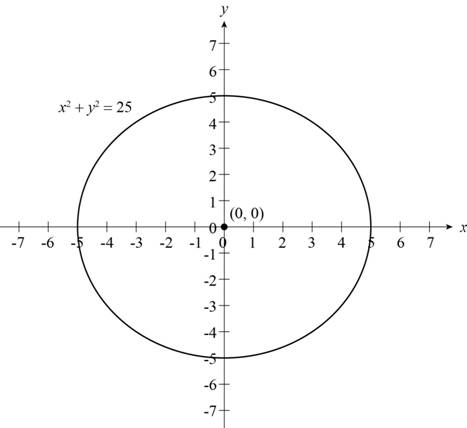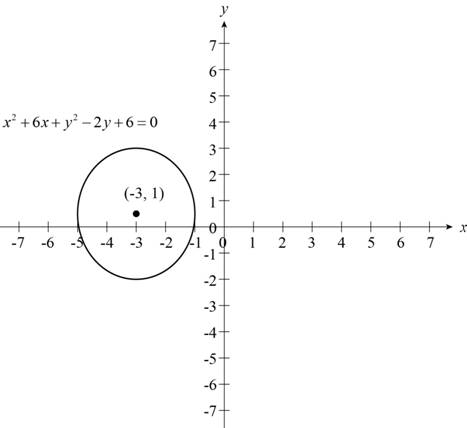# The center and radius of circle x 2 + y 2 = 25 and draw its graph.### Precalculus: Mathematics for Calcu...

6th Edition
Stewart + 5 others
Publisher: Cengage Learning
ISBN: 9780840068071### Precalculus: Mathematics for Calcu...

6th Edition
Stewart + 5 others
Publisher: Cengage Learning
ISBN: 9780840068071

#### Solutions

Chapter 1, Problem 18T

(a)

To determine

## To find: The center and radius of circle x2+y2=25 and draw its graph.

Expert Solution

The center of circle is (0,0) and radius of circle is 5.

### Explanation of Solution

Section1:

The given equation of circle is x2+y2=25

The equation of circle with center (0,0) and radius r is,

x2+y2=r2 . (1)

Rewrite the given equation,

x2+y2=(5)2

Compare above equation with equation (1) to get the center (0,0) and radius r=5 of circle.

Thus, the center of circle is (0,0) and radius of circle is 5.

Section2:

From the section1 the center of circle is pass through origin and the radius of circle is 5 that is shown below,Figure (1)

Figure (1) shows the graph of circle x2+y2=25 with center (0,0) and radius 5.

(b)

To determine

### To find: The center and radius of circle (x−2)2+(y+1)2=9 and draw its graph.

Expert Solution

The center of circle is (2,1) and radius of circle is 3.

### Explanation of Solution

Section1:

The given equation of circle is (x2)2+(y+1)2=9

The equation of circle with center (h,k) and radius r is,

(xh)2+(yk)2=r2 . (1)

Rewrite the given equation,

(x2)2+(y+1)2=(3)2

Compare given equation with above equation to get the center (2,1) and radius r=3 of circle.

Thus, the center of circle is (2,1) and radius of circle is 3.

Section2:

The given equation of circle is (x2)2+(y+1)2=9 .

From the section1 the center of circle is (2,1) and the radius of circle is 3 that is shown below,Figure (2)

Figure (2) shows the graph of circle (x2)2+(y+1)2=9 with center (2,1) and radius 3.

(c)

To determine

### To find: The center and radius of circle x2+6x+y2−2y+6=0 and draw its graph.

Expert Solution

The center of circle is (3,1) and radius of circle is 2.

### Explanation of Solution

Given:

The equation is x2+6x+y22y+6=0 .

Calculation:

Section1:

The equation of circle with center (h,k) and radius r is,

(xh)2+(yk)2=r2 . (1)

First, group the x-terms and y-terms then make a complete square within each group in the given equation,

(x2+6x)+(y22y)=6

Add (126)2=9 to complete the square of (x2+6x) and (12(2))2=1 to complete the square of (y22y) and solve for center and radius of circle,

(x2+6x+9)+(y22y+1)=6+9+1(x2+2x3+(3)2)+(y22y1+(1)2)=4(x+3)2+(y1)2=(2)2

Compare the above equation with equation (1) to get the center (3,1) and radius r=2 of circle.

Thus, the center of circle is (3,1) and radius of circle is 2.

Section2:

From the section1 the center of circle is (3,1) and the radius of circle is 2 that is shown below,Figure (3)

Figure (3) shows the graph of circle x2+6x+y22y+6=0 with center (2,1) and radius 2.

### Have a homework question?

Subscribe to bartleby learn! Ask subject matter experts 30 homework questions each month. Plus, you’ll have access to millions of step-by-step textbook answers!